Examples

Chapter 3 Class 9 Coordinate Geometry
Serial order wise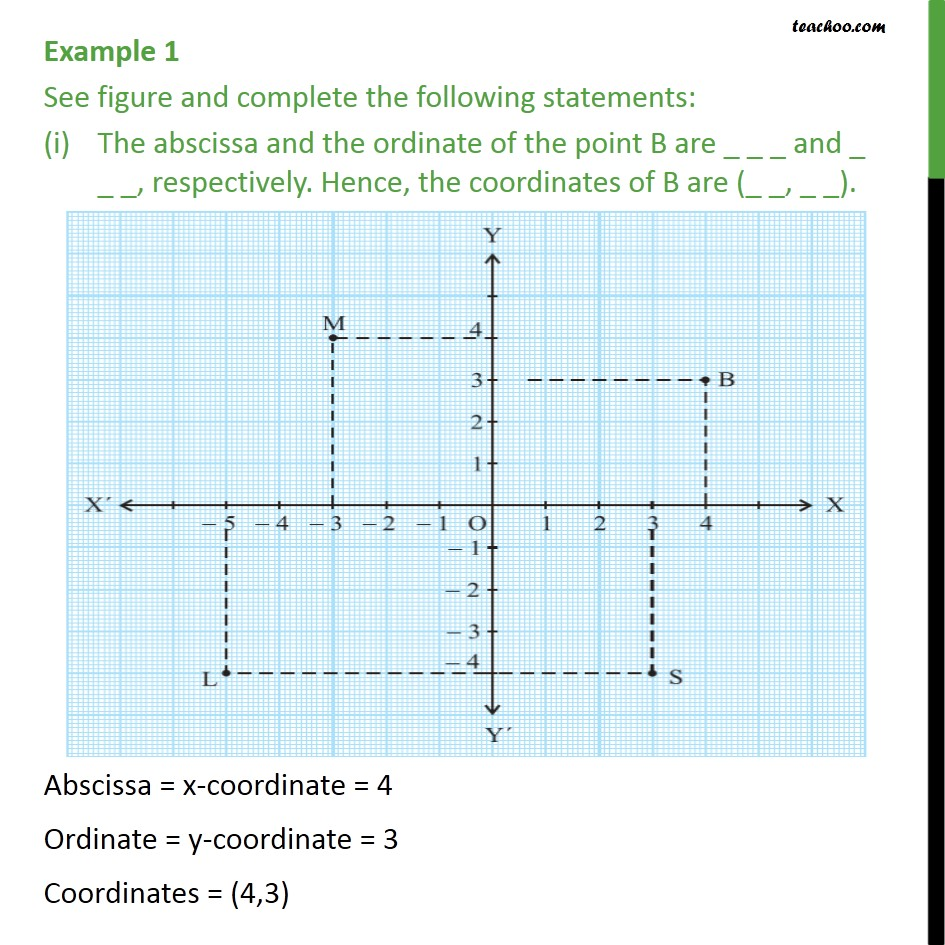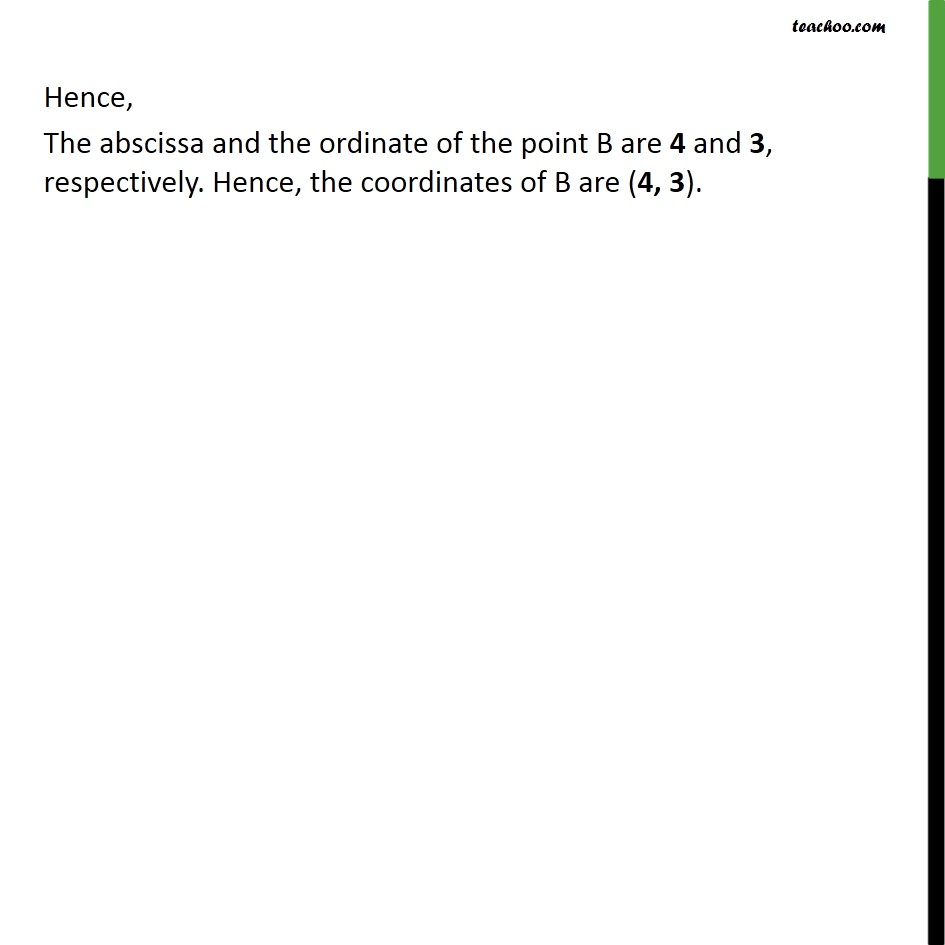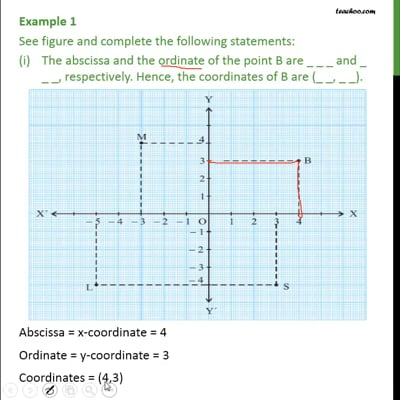This video is only available for Teachoo black users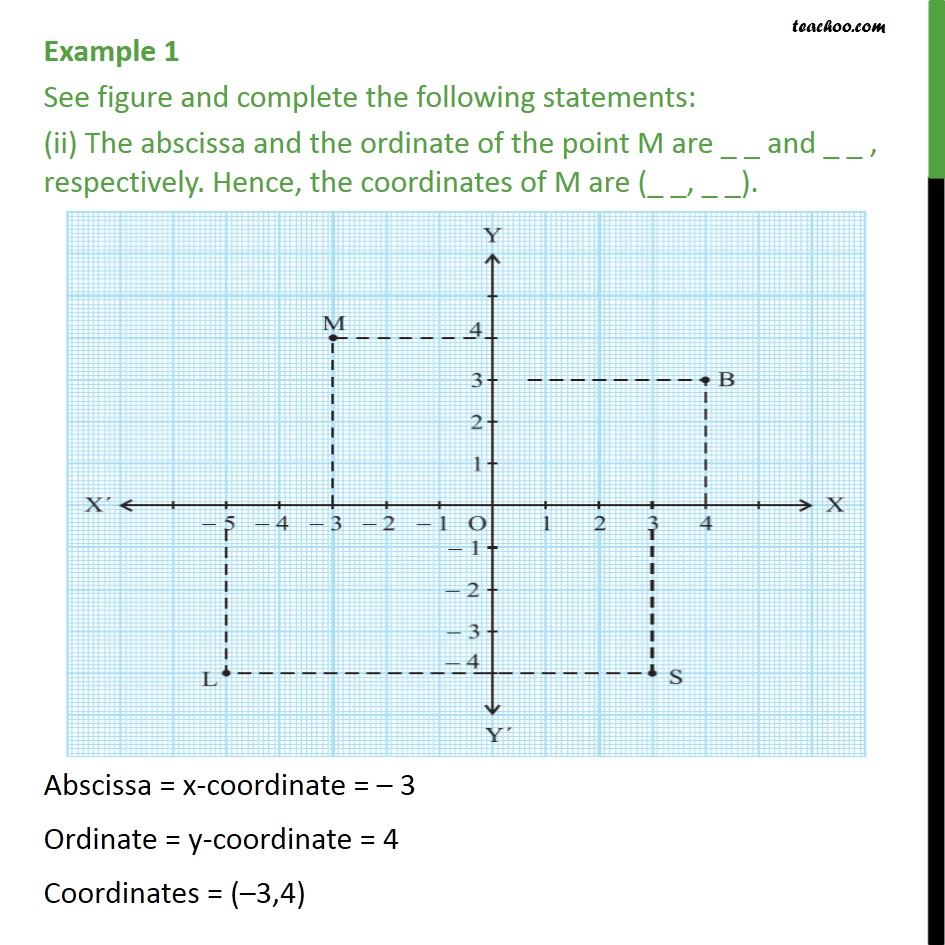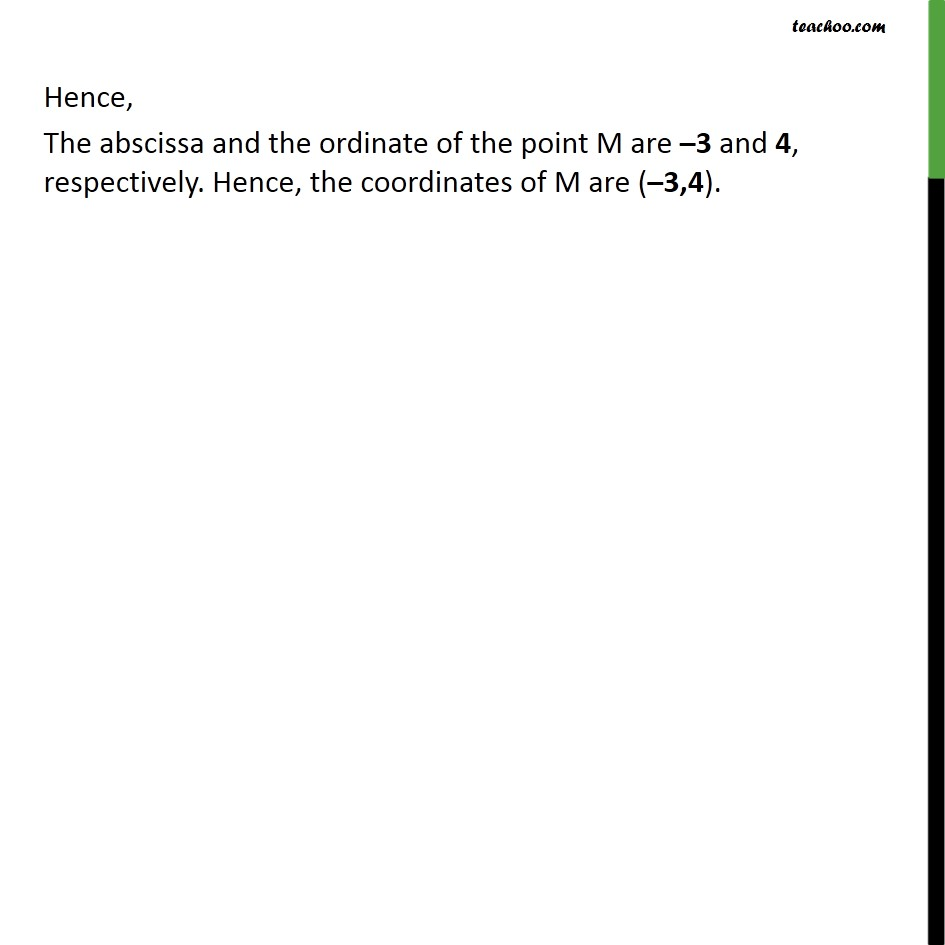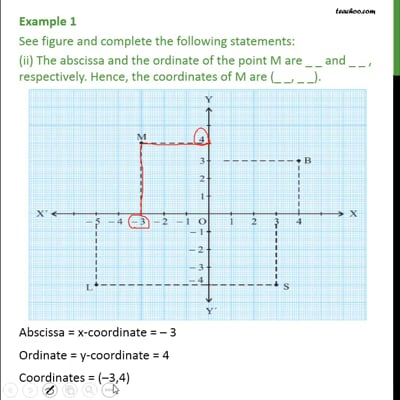This video is only available for Teachoo black users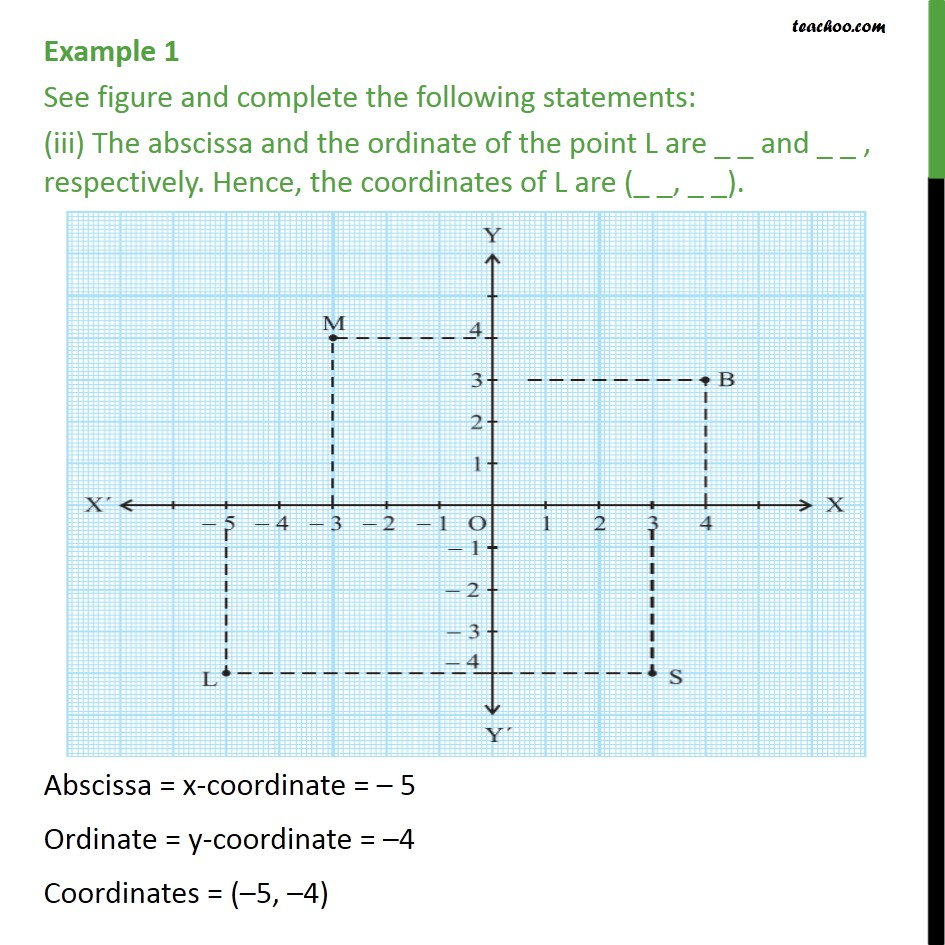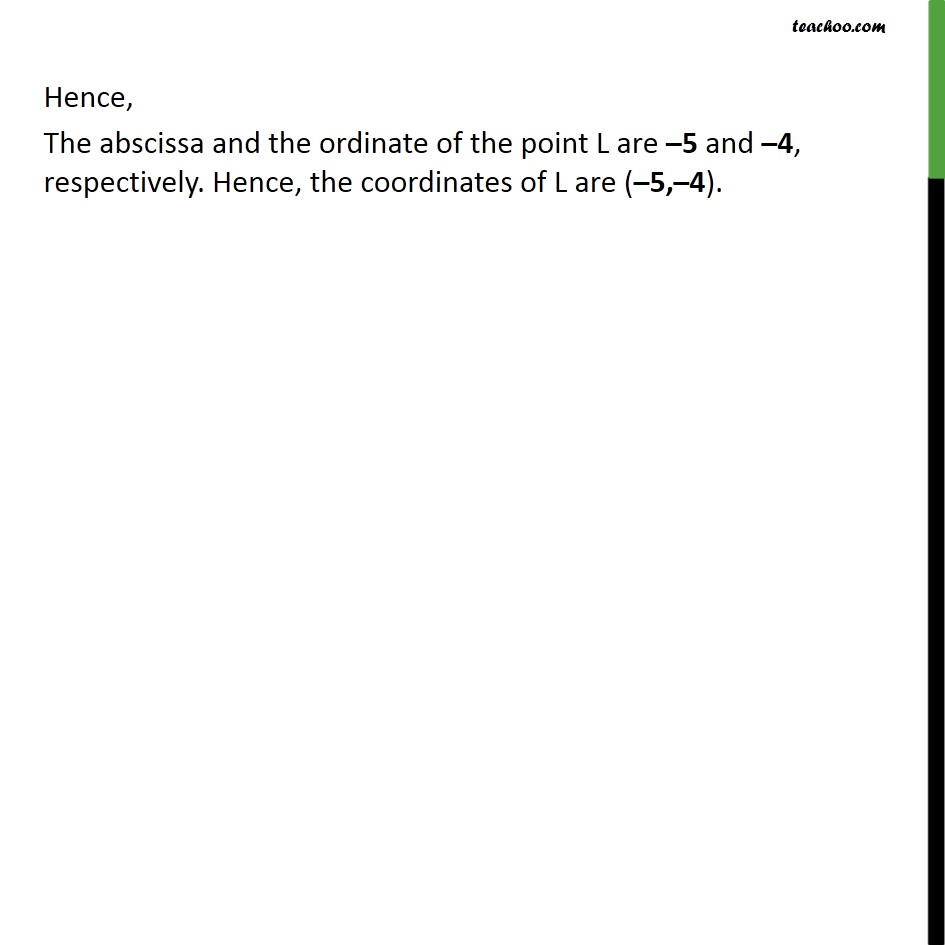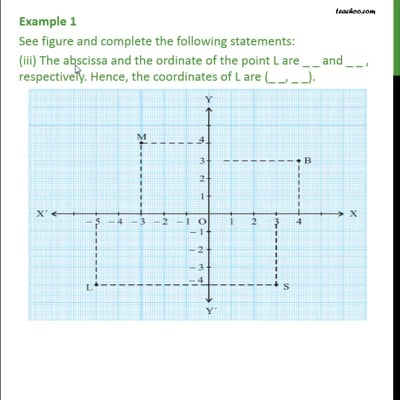This video is only available for Teachoo black users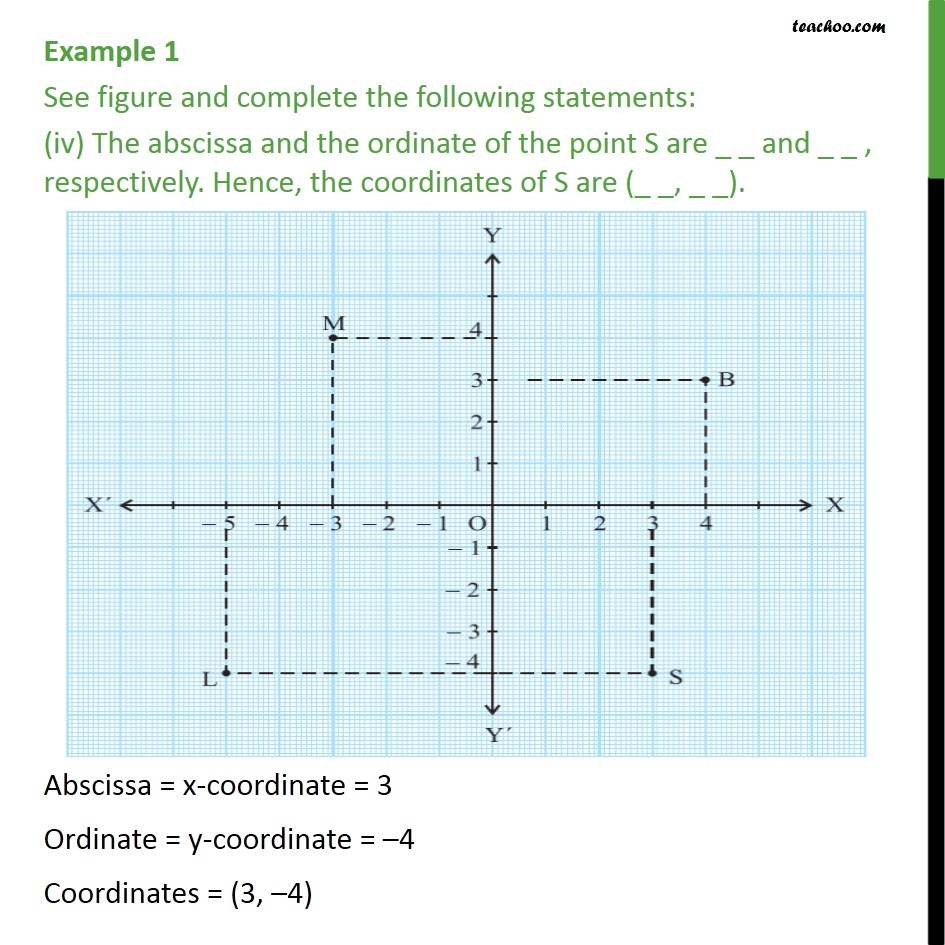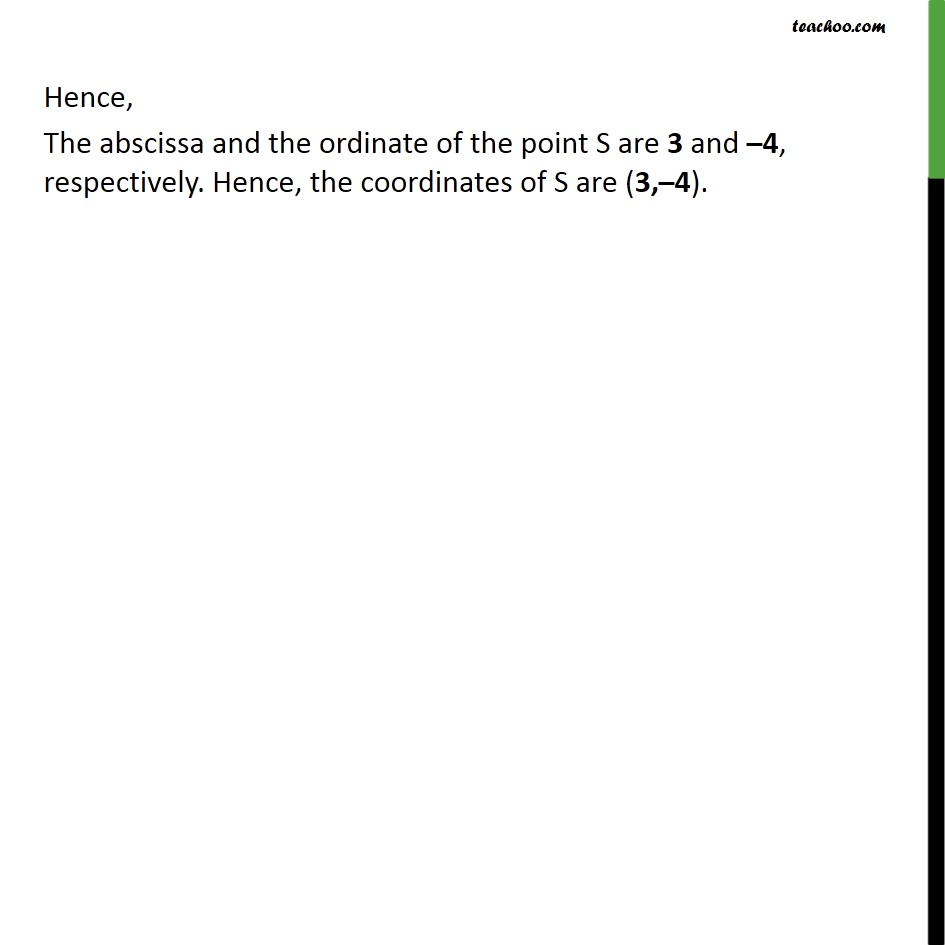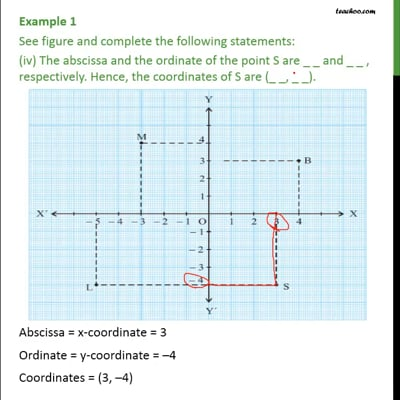This video is only available for Teachoo black users

Learn in your speed, with individual attention - Teachoo Maths 1-on-1 Class

### Transcript

Example 1 See figure and complete the following statements: The abscissa and the ordinate of the point B are _ _ _ and _ _ _, respectively. Hence, the coordinates of B are (_ _, _ _). Abscissa = x-coordinate = 4 Ordinate = y-coordinate = 3 Coordinates = (4,3) Hence, The abscissa and the ordinate of the point B are 4 and 3, respectively. Hence, the coordinates of B are (4, 3). Example 1 See figure and complete the following statements: (ii) The abscissa and the ordinate of the point M are _ _ and _ _ , respectively. Hence, the coordinates of M are (_ _, _ _). Abscissa = x-coordinate = – 3 Ordinate = y-coordinate = 4 Coordinates = (–3,4) Hence, The abscissa and the ordinate of the point M are –3 and 4, respectively. Hence, the coordinates of M are (–3,4). Example 1 See figure and complete the following statements: (iii) The abscissa and the ordinate of the point L are _ _ and _ _ , respectively. Hence, the coordinates of L are (_ _, _ _). Abscissa = x-coordinate = – 5 Ordinate = y-coordinate = –4 Coordinates = (–5, –4) Hence, The abscissa and the ordinate of the point L are –5 and –4, respectively. Hence, the coordinates of L are (–5,–4). Example 1 See figure and complete the following statements: (iv) The abscissa and the ordinate of the point S are _ _ and _ _ , respectively. Hence, the coordinates of S are (_ _, _ _). Abscissa = x-coordinate = 3 Ordinate = y-coordinate = –4 Coordinates = (3, –4) Hence, The abscissa and the ordinate of the point S are 3 and –4, respectively. Hence, the coordinates of S are (3,–4).